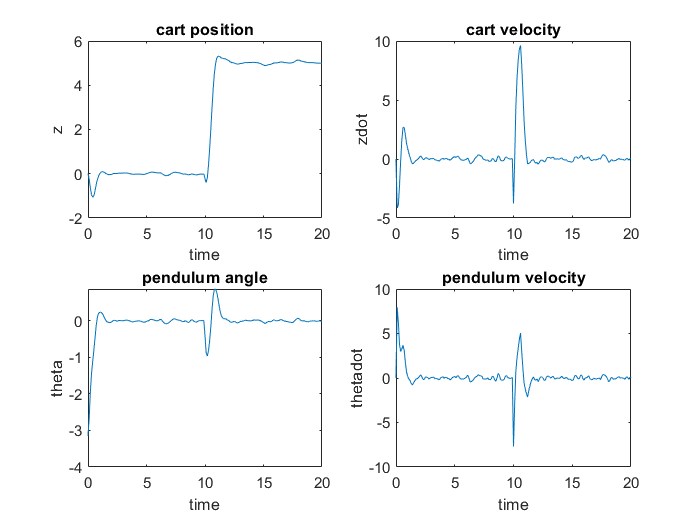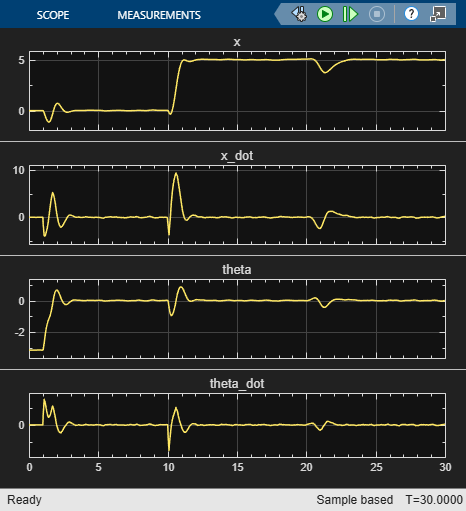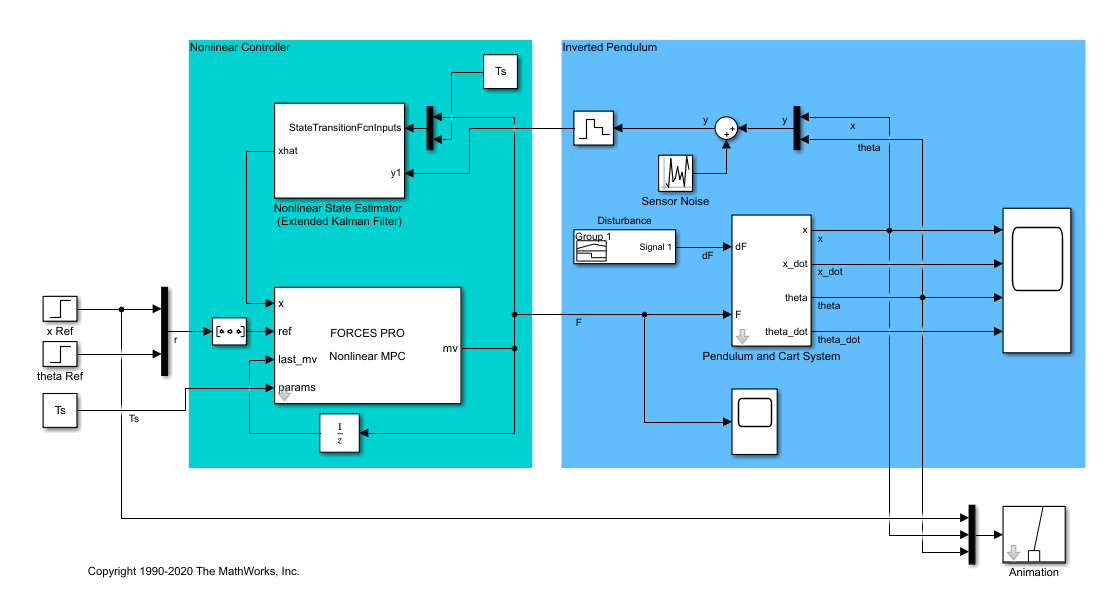# Swing-up Control of a Pendulum Using Nonlinear Model Predictive Control

This example uses a nonlinear model predictive controller object and block to achieve swing-up and balancing control of an inverted pendulum on a cart.

This example requires Optimization Toolbox™ software to provide the default nonlinear programming solver for nonlinear MPC to compute optimal control moves at each control interval.

### Pendulum/Cart Assembly

The plant for this example is a pendulum/cart assembly, where z is the cart position and theta is the pendulum angle. The manipulated variable for this system is a variable force F acting on the cart. The range of the force is between `-100` and `100`. An impulsive disturbance dF can push the pendulum as well.### Control Objectives

Assume the following initial conditions for the pendulum/cart assembly.

• The cart is stationary at z = `0`.

• The pendulum is in a downward equilibrium position where theta = `-pi`.

The control objectives are:

• Swing-up control: Initially swing the pendulum up to an inverted equilibrium position where z = `0` and theta = `0`.

• Cart position reference tracking: Move the cart to a new position with a step setpoint change, keeping the pendulum inverted.

• Pendulum balancing: When an impulse disturbance of magnitude of `2` is applied to the inverted pendulum, keep the pendulum balanced, and return the cart to its original position.

The downward equilibrium position is stable, and the inverted equilibrium position is unstable, which makes swing-up control more challenging for a single linear controller, which nonlinear MPC handles easily.

### Control Structure

In this example, the nonlinear MPC controller has the following I/O configuration.

• One manipulated variable: Variable force (F)

• Two measured outputs: Cart position (z) and pendulum angle (theta)

Two other states, cart velocity (zdot) and pendulum angular velocity (thetadot) are not measurable.

While the setpoint of the cart position, z, can vary, the setpoint of the pendulum angle, theta, is always `0` (inverted equilibrium position).

### Create Nonlinear MPC Controller

Create a nonlinear MPC controller with the proper dimensions using an `nlmpc` object. In this example, the prediction model has `4` states, `2` outputs, and `1` input (MV).

```nx = 4; ny = 2; nu = 1; nlobj = nlmpc(nx, ny, nu); ```
```In standard cost function, zero weights are applied by default to one or more OVs because there are fewer MVs than OVs. ```

The prediction model has a sample time of `0.1` seconds, which is the same as the controller sample time.

```Ts = 0.1; nlobj.Ts = Ts; ```

Set the prediction horizon to `10`, which is long enough to capture major dynamics in the plant but not so long that it hurts computational efficiency.

```nlobj.PredictionHorizon = 10; ```

Set the control horizon to `5`, which is long enough to give the controller enough degrees of freedom to handle the unstable mode without introducing excessive decision variables.

```nlobj.ControlHorizon = 5; ```

### Specify Nonlinear Plant Model

The major benefit of nonlinear model predictive control is that it uses a nonlinear dynamic model to predict plant behavior in the future across a wide range of operating conditions.

This nonlinear model is usually a first principle model consisting of a set of differential and algebraic equations (DAEs). In this example, a discrete-time cart and pendulum system is defined in the `pendulumDT0` function. This function integrates the continuous-time model, `pendulumCT0`, between control intervals using a multistep forward Euler method. The same function is also used by the nonlinear state estimator.

```nlobj.Model.StateFcn = "pendulumDT0"; ```

To use a discrete-time model, set the `Model.IsContinuousTime` property of the controller to `false`.

```nlobj.Model.IsContinuousTime = false; ```

The prediction model uses an optional parameter, `Ts`, to represent the sample time. Using this parameter means that, if you change the prediction sample time during the design, you do not have to modify the `pendulumDT0` file.

```nlobj.Model.NumberOfParameters = 1; ```

The two plant outputs are the first and third state in the model, the cart position and pendulum angle, respectively. The corresponding output function is defined in the `pendulumOutputFcn` function.

```nlobj.Model.OutputFcn = 'pendulumOutputFcn'; ```

It is best practice to provide analytical Jacobian functions whenever possible, since they significantly improve the simulation speed. In this example, provide a Jacobian for the output function using an anonymous function.

```nlobj.Jacobian.OutputFcn = @(x,u,Ts) [1 0 0 0; 0 0 1 0]; ```

Since you do not provide Jacobian for the state function, the nonlinear MPC controller estimates the state function Jacobian during optimization using numerical perturbation. Doing so slows down simulation to some degree.

### Define Cost and Constraints

Like linear MPC, nonlinear MPC solves a constrained optimization problem at each control interval. However, since the plant model is nonlinear, nonlinear MPC converts the optimal control problem into a nonlinear optimization problem with a nonlinear cost function and nonlinear constraints.

The cost function used in this example is the same standard cost function used by linear MPC, where output reference tracking and manipulated variable move suppression are enforced. Therefore, specify standard MPC tuning weights.

```nlobj.Weights.OutputVariables = [3 3]; nlobj.Weights.ManipulatedVariablesRate = 0.1; ```

The cart position is limited to the range `-10` to `10`.

```nlobj.OV(1).Min = -10; nlobj.OV(1).Max = 10; ```

The force has a range between `-100` and `100`.

```nlobj.MV.Min = -100; nlobj.MV.Max = 100; ```

### Validate Nonlinear MPC Controller

After designing a nonlinear MPC controller object, it is best practice to check the functions you defined for the prediction model, state function, output function, custom cost, and custom constraints, as well as their Jacobians. To do so, use the `validateFcns` command. This function detects any dimensional and numerical inconsistencies in these functions.

```x0 = [0.1;0.2;-pi/2;0.3]; u0 = 0.4; validateFcns(nlobj,x0,u0,[],{Ts}); ```
```Model.StateFcn is OK. Model.OutputFcn is OK. Jacobian.OutputFcn is OK. Analysis of user-provided model, cost, and constraint functions complete. ```

### State Estimation

In this example, only two plant states (cart position and pendulum angle) are measurable. Therefore, you estimate the four plant states using an extended Kalman filter. Its state transition function is defined in `pendulumStateFcn.m` and its measurement function is defined in `pendulumMeasurementFcn.m`.

```EKF = extendedKalmanFilter(@pendulumStateFcn, @pendulumMeasurementFcn); ```

### Closed-Loop Simulation in MATLAB®

Specify the initial conditions for simulations by setting the initial plant state and output values. Also, specify the initial state of the extended Kalman filter.

The initial conditions of the simulation areas follows.

• The cart is stationary at z = 0.

• The pendulum is in a downward equilibrium position, theta = `-pi`.

```x = [0;0;-pi;0]; y = [x(1);x(3)]; EKF.State = x; ```

`mv` is the optimal control move computed at any control interval. Initialize `mv` to zero, since the force applied to the cart is zero at the beginning.

```mv = 0; ```

In the first stage of the simulation, the pendulum swings up from a downward equilibrium position to an inverted equilibrium position. The state references for this stage are all zero.

```yref1 = [0 0]; ```

At a time of `10` seconds, the cart moves from position `0` to `5`. Set the state references for this position.

```yref2 = [5 0]; ```

Using the `nlmpcmove` command, compute optimal control moves at each control interval. This function constructs a nonlinear programming problem and solves it using the `fmincon` function from the Optimization Toolbox.

Specify the prediction model parameter using an `nlmpcmoveopt` object, and pass this object to `nlmpcmove`.

```nloptions = nlmpcmoveopt; nloptions.Parameters = {Ts}; ```

Run the simulation for `20` seconds.

```Duration = 20; hbar = waitbar(0,'Simulation Progress'); xHistory = x; for ct = 1:(20/Ts) % Set references if ct*Ts<10 yref = yref1; else yref = yref2; end % Correct previous prediction using current measurement. xk = correct(EKF, y); % Compute optimal control moves. [mv,nloptions,info] = nlmpcmove(nlobj,xk,mv,yref,[],nloptions); % Predict prediction model states for the next iteration. predict(EKF, [mv; Ts]); % Implement first optimal control move and update plant states. x = pendulumDT0(x,mv,Ts); % Generate sensor data with some white noise. y = x([1 3]) + randn(2,1)*0.01; % Save plant states for display. xHistory = [xHistory x]; %#ok<*AGROW> waitbar(ct*Ts/20,hbar); end close(hbar) ```

Plot the closed-loop response.

```figure subplot(2,2,1) plot(0:Ts:Duration,xHistory(1,:)) xlabel('time') ylabel('z') title('cart position') subplot(2,2,2) plot(0:Ts:Duration,xHistory(2,:)) xlabel('time') ylabel('zdot') title('cart velocity') subplot(2,2,3) plot(0:Ts:Duration,xHistory(3,:)) xlabel('time') ylabel('theta') title('pendulum angle') subplot(2,2,4) plot(0:Ts:Duration,xHistory(4,:)) xlabel('time') ylabel('thetadot') title('pendulum velocity') ```The pendulum angle plot shows that the pendulum successfully swings up in two seconds. During the swing-up process, the cart is displaced with a peak deviation of `-1`, and returned to its original position around a time of `2` seconds.

The cart position plot shows that the cart successfully moves to z = `5` in two seconds. While the cart moves, the pendulum is displaced with a peak deviation of `1` radian (`57` degrees) and returned to an inverted equilibrium position around a time of `12` seconds.

### Closed-Loop Simulation with FORCESPRO Solver in MATLAB

You can easily use a third-party nonlinear programming solver together with the nonlinear MPC object designed using Model Predictive Control Toolbox software. For example, if you have FORCESPRO software from Embotech installed, you can use their MPC Toolbox Plugin to generate an efficient custom NLP solver from your `nlmpc` object and use the solver for simulation and code generation.

First, generate a custom solver using the `nlmpcToForces` command. You can choose using either an Interior-Point (IP) solver or a Sequential Quadratic Programming (SQP) solver using the `nlmpcToForcesOptions` command.

```options = nlmpcToForcesOptions(); options.SolverName = 'MyIPSolver'; options.SolverType = 'InteriorPoint'; options.Parameter = Ts; options.x0 = [0;0;-pi;0]; options.mv0 = 0; [coredata, onlinedata] = nlmpcToForces(nlobj,options); ```

The `nlmpcToForces` function generates a custom MEX function `nlmpcmove_MyIPSolver`, which you can use to speed up closed-loop simulation.

```x = [0;0;-pi;0]; mv = 0; EKF.State = x; y = [x(1);x(3)]; hbar = waitbar(0,'Simulation Progress'); xHistory = x; for ct = 1:(20/Ts) % Set references if ct*Ts<10 onlinedata.ref = repmat(yref1,10,1); else onlinedata.ref = repmat(yref2,10,1); end % Correct previous prediction using current measurement. xk = correct(EKF, y); % Compute optimal control moves using FORCESPRO solver. [mv,onlinedata,info] = nlmpcmove_MyIPSolver(xk,mv,onlinedata); % Predict prediction model states for the next iteration. predict(EKF, [mv; Ts]); % Implement first optimal control move and update plant states. x = pendulumDT0(x,mv,Ts); % Generate sensor data with some white noise. y = x([1 3]) + randn(2,1)*0.01; % Save plant states for display. xHistory = [xHistory x]; %#ok<*AGROW> waitbar(ct*Ts/20,hbar); end close(hbar) ```

As expected, the closed-loop response is similar to the one obtained using `fmincon` as expected.Validate the nonlinear MPC controller with a closed-loop simulation in Simulink.

```mdl = 'mpc_pendcartNMPC'; open_system(mdl) ```In this model, the Nonlinear MPC Controller block is configured to use the previously designed controller, `nlobj`.

To use optional parameters in the prediction model, the model has a Simulink Bus block connected to the `params` input port of the Nonlinear MPC Controller block. To configure this bus block to use the `Ts` parameter, create a Bus object in the MATLAB workspace and configure the Bus Creator block to use this object. To do so, use the `createParameterBus` function. In this example, name the Bus object `'myBusObject'`.

```createParameterBus(nlobj,[mdl '/Nonlinear MPC Controller'],'myBusObject',{Ts}); ```
```A Simulink Bus object "myBusObject" created in the MATLAB Workspace, and Bus Creator block "mpc_pendcartNMPC/Nonlinear MPC Controller" is configured to use it. ```

Run the simulation for `30` seconds.

```open_system([mdl '/Scope']) sim(mdl) ```The nonlinear simulation in Simulink produces identical swing-up and cart position tracking results compared to the MATLAB simulation. Also, a push (impulse disturbance `dF`) is applied to the inverted pendulum at a time of `20` seconds. The nonlinear MPC controller successfully rejects the disturbance and returns the cart to z = `5` and the pendulum to an inverted equilibrium position.

### Closed-Loop Simulation with FORCESPRO Solver in Simulink

You can also use the FORCES Nonlinear MPC block from the Embotech FORCESPRO software to simulate the nonlinear MPC using the generated custom NLP solver.

If you have FORCESPRO software installed, open the `mpc_pendcartFORCESPRO` model and run it to completion. The closed-loop response is similar to the one using `fmincon`.### Conclusion

This example illustrates a general workflow to design and simulate nonlinear MPC in MATLAB and Simulink using an `nlmpc` object and Nonlinear MPC Controller block, respectively. Depending on the specific nonlinear plant characteristics and control requirements, the implementation details can vary significantly. The key design challenges include:

• Choosing proper horizons, bounds, and weights

• Designing a nonlinear state estimator

• Designing a custom nonlinear cost function and constraint function

• Selecting solver options or choosing a custom NLP solver

You can use the functions and Simulink model in this example as templates for other nonlinear MPC design and simulation tasks.

Both the `nlmpcmoveCodeGeneration` command from Model Predictive Control Toolbox software and the `nlmpcmoveForces` command from FORCESPRO support code generation in MATLAB. Both the Nonlinear MPC block from Model Predictive Control Toolbox software and `FORCES Nonlinear MPC` block from FORCESPRO support code generation in Simulink.